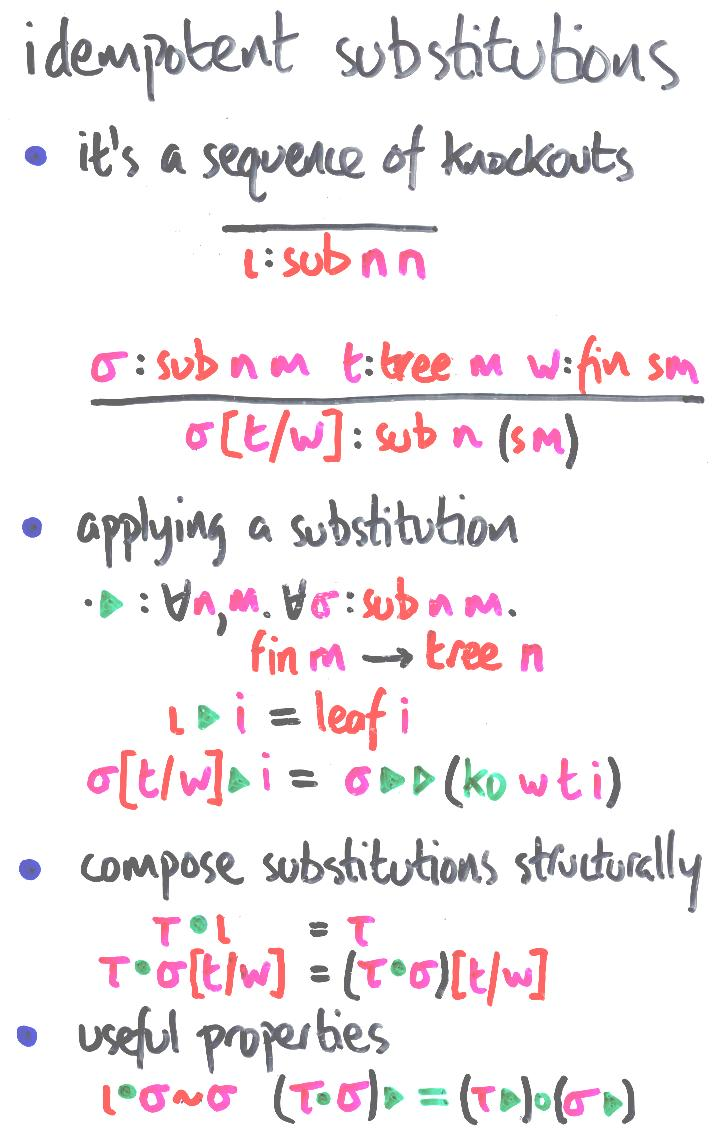Next: embeddings | Prev: accumulating a unifier | Up: contentsThis is a concrete datatype representing substitutions which are idempotent in the same sense that lightning never strikes the same place twice: the same place isn't there twice. This datatype does not capture all the idempotent substitutions, because it does not permit permutations of variables. It does contain enough.

The type sub n m is interpreted as that of substitutions from m variables to terms with n variables. (I like my sources on the right.)

The two constructors are interpreted, respectively, as

• the identity substitution
• the extension of sigma (at the front) with a knockout: t for w

I apologise for not writing

• iota >> = leaf
sigma[t/w] >> = (sigma >>) o (ko t w)
(where >> is lazy html for the solid triangle)

>> embeds these substitutions into the functional presentation of simultaneous substitutions. The meaning of sigma extended by t for w is `first knock out w by t, then do sigma to the result'.

Experienced typespotters will recognise sub n m as a cross between an association list and the `suffix' version of n <= m.

There are two standard presentations of <= for nat:

• suffix:

-----------------------
n <= n

n <= m
-----------------------
n <= sm

• prefix:

-----------------------
0 <= m

n <= m
-----------------------
sn <= sm

Think `list' to understand the names. The only reason why these both code up the same relation is the deceptive symmetry of the natural numbers. (I suspect God invented the natural numbers especially to cause us this kind of confusion.)

The obvious `subtractive' way to write a <= function corresponds to the prefix version. The suffix version involves fooling about with equality tests. (In fact, I prefer the suffix version, because it generalises to the subterm relation/test for less symmetrical datatypes, and is easily proven transitive: it's hard to see how prefix generalises to datatypes without a useful notion of zero.) I don't think there's a sensible prefix version of sub.

I mention this because I'm trying to figure out how much you have to pay for this datatype if you have to compute it from the indices, rather than using dependently typed constructors as LEGO permits. The jury's out.

There's an obvious syntactic composition for sub, combining transitivity for <= and append for a-lists. Extensionally, at least, everything behaves as it should.

Exercise: this sub is the one-at-a-time version; try the normalised version.

Next: embeddings | Prev: accumulating a unifier | Up: contents

Conor's Work Page